Function Repository Resource:

# ValuesReduceOperator

Group data according to the positionally corresponding values of other data

Contributed by: Seth J. Chandler
 ResourceFunction["ValuesReduceOperator"][] creates a pure function that will group the data in its first argument according to the value in the same position of the second argument. ResourceFunction["ValuesReduceOperator"][h] reduces the groups of data by applying the function h to each of the groups.

## Details

ResourceFunction["ValuesReduceOperator"] is similar to the resource function MapReduceOperator, except that instead of the grouping being done based on a function of the data, the grouping is done based on pre-computed values, which become the keys to the Association produced by the function.

## Examples

### Basic Examples (2)

Group a four-membered list of pairs according to the values of a four-membered list:

 In:=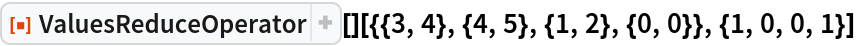Out=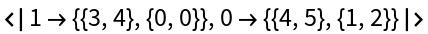Group a four member list of pairs according to the values of a four member list and produce a MatrixPlot of each grouping:

 In:=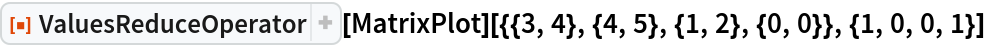Out=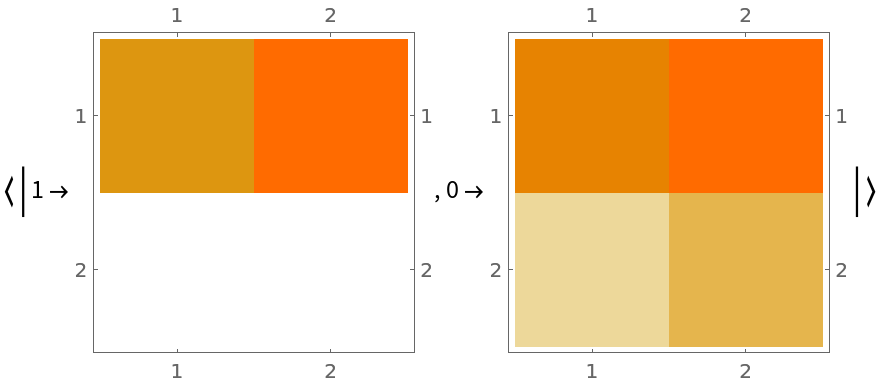### Scope (2)

The data to be grouped can be anything:

 In:=Out=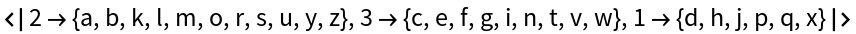The values can be anything:

 In:=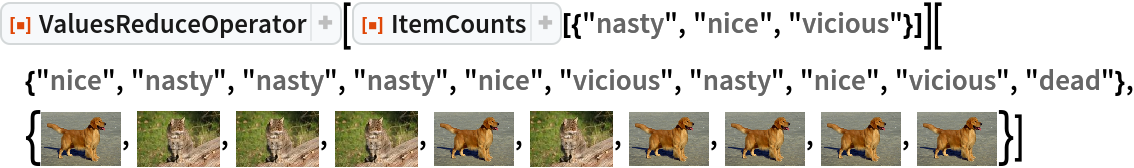Out=### Applications (3)

A feature matrix and a target vector:

 In:=Plot the features color-coded by the targets:

 In:=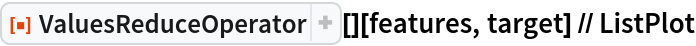Out=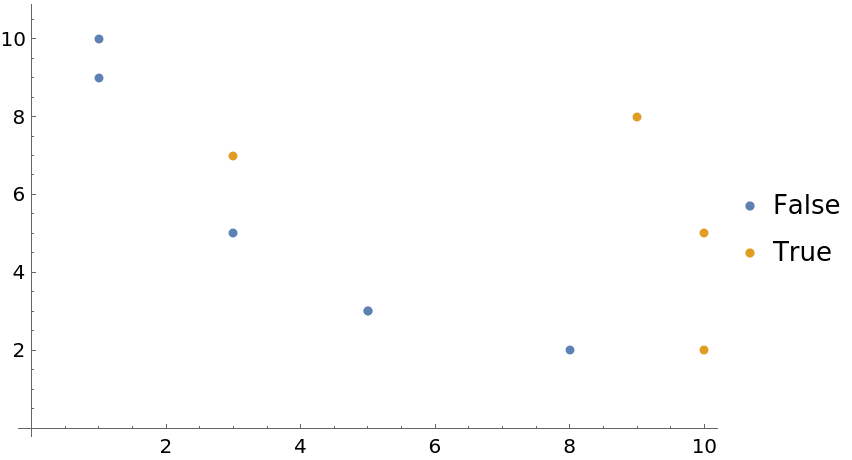Show the mean value of features grouped by the value of the target:

 In:=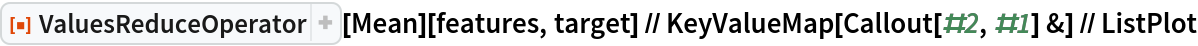Out=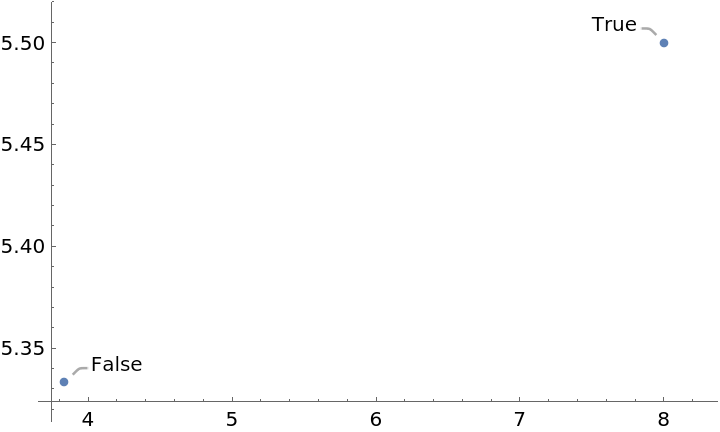Seth J. Chandler

## Version History

• 1.0.0 – 24 March 2021